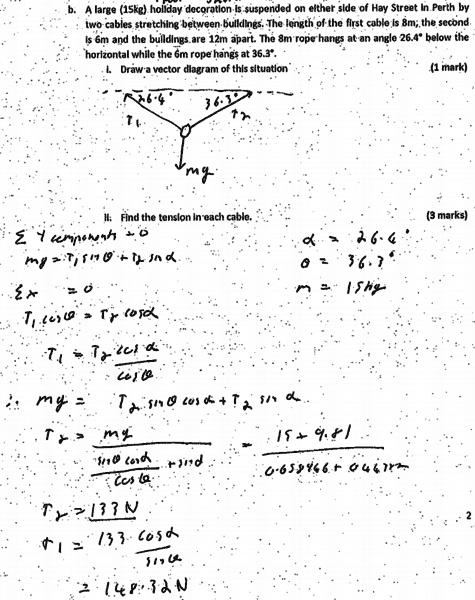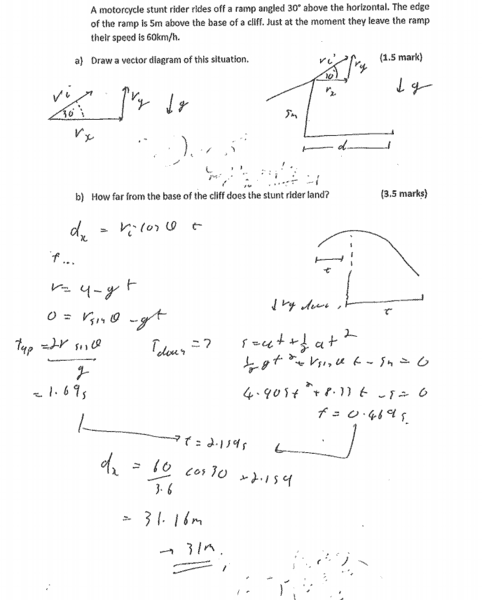# Solution verification for various problems

I have solutions to some physics problems that have been given to me which I think may have errors in them. I would appreciate somebody checking them for me as I am not confident enough to question them without someone more experienced checking them first.

Here's one of them. (I believe the trigonometry is incorrect)(Apologies for the bad quality of the document -- this was exactly how I was given the paper.

#### Attachments

Simon Bridge
Homework Helper
The way to check someone elses work is to figure out a model answer yourself. How would you do it?
Compare with what they did - see if you can follow their reasoning.

1. I'd have preferred the horizontal dotted line drawn through the mass for a FBD, but I wouldn't deduct marks for what was done there.

2. I'd have liked to see the initial equations numbered and the words "2 simultaneous equations, 2 unknowns" there someplace, then the numbers used to explain the substitution.

After the "therefore" I think there should be a cosine in the quotient - it materialized from nowhere in the next line. Note: sin/cos = tan.

The full answer should finish off writing the results in terms used by the question:
"The tension in the 6m cable is... the tension in the 8m cable is ..." like that.

There's three marks allocated for Q2, so there'd be 1 for the force equations, 1 for the substitution, and 1 for working the solution. I'd mark down 0.5 (if that) for the messed derivation (unclear).

Total 4.5/5 (worst case).
OTOH: I never give marks for the correct number out the end, so I didn't bother checking that. I mean: it is important to be able to punch numbers into a calculator/computer properly, I just don't think it's worth marks.

In general, if you do work this good, try to be clear: you can only win.
Assume the person marking your work has just got through 200 like it, your's is the last one, it's 2am, and their lover is calling from the bedroom ... do they stop to decide whether to give you that 0.5 mark or just leave it and go to bed? Make it easy for them to decide in your favour :)

Last edited:
1. I'd have preferred the horizontal dotted line drawn through the mass for a FBD, but I wouldn't deduct marks for what was done there.

2. I'd have liked to see the initial equations numbered and the words "2 simultaneous equations, 2 unknowns" there someplace, then the numbers used to explain the substitution.

After the "therefore" I think there should be a cosine in the quotient - it materialized from nowhere in the next line. Note: sin/cos = tan.

There's three marks allocated for that, so there'd be 1 for the force equations, 1 for the substitution, and 1 for working the solution. I'd mark down 0.5 (if that) for the messed derivation (unclear).

Total 4.5/5 (worst case).
OTOH: I never give marks for the correct number out the end, so I didn't bother checking that. I mean: it is important to be able to punch numbers into a calculator/computer properly, I just don't think it's worth marks.

So with regards to this equation:
mg = T1 sin Ɵ + T2 sin α

shouldn't the equation be T2sin Ɵ + T1sin α
because it appears the hypotenuse for both parts of the equation has been swapped -- i.e. shouldn't it be that T1 is the hypotenuse for α and the hypotenuse for T2 is Ɵ?

Also, with the second equation, I was thinking it too has the same issue with the respective hyptonuses being swapped over.

Last edited:
Simon Bridge
Homework Helper
Well spotted - yep.

Or that you want to swap theta and alpha over in the key :) Check the numbers to see if they have managed to use the correct angle with the correct tension.
(checked - nah they didn't)

their value for sin(\alpha) looks like 0.447something and I get 0.44464 - may want to check the number-crunching completely then.

Would we drop a whole mark for blowing the derivation, though their reasoning was sound?
That would be 3.5/5

Last edited:
Well spotted - yep.

Or that you want to swap theta and alpha over in the key :) Check the numbers to see if they have managed to use the correct angle with the correct tension.
(checked - nah they didn't)

their value for sin(\alpha) looks like 0.447something and I get 0.44464 - may want to check the number-crunching completely then.

Would we drop a whole mark for blowing the derivation, though their reasoning was sound?
That would be 3.5/5

I had another problem that I was wishing to verify:Has he gone about this problem correctly, or are there any subtle mistakes here too (e.g. with the trigonometry again)?

Simon Bridge